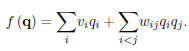# Laminar Flow on D-Wave

Hi guys,

I am trying to implement a solver on a D-Wave quantum computer for a 1-dimensional laminar flow. Now just trying to repeat the work done by N.Ray et al: https://arxiv.org/abs/1904.09033 (for a classical computer this problem is quite easy one).

Basically, pseudocode provided in the paper consists of following subroutines:

1) construct_linear_system

2) convert_to_fixed_point

3) construct_right_hand_side

4) construct_qubo_matrix

5) solve_qubo

6) convert_binary_to_real

7) weighted_average

I already implemented most of the subroutines: 1), 2), 3), 6), and partly 4).

My code is (it is pretty simple) is here https://github.com/aiskhak/aiskhak.

a) I have problems with construct_qubo_matrix. To do it it is neccessary to use formulaI calculated v_i and w_i,j but I do not understand what to do next (how to put it in QC).

b) how to solve this problem on D-Wave - solve_qubo subroutine?

c) how to average output values using  weighted_average?

I would be very happy to get your help.

Thank you.

• Looks like I got some results. Just want to clarify something:

1) does the exact solver ExactSolver().sample(bqm) gives exact solution?

2) if so, why there are so many responses (with different energies)?

• Hello,

The ExactSolver is a brute force solver that gives you every possible combination of variable values.

The results correspond to all of these combinations, and they are ordered by energy, lowest to highest.

It does not do well as the number of variables go up, but it is good for smaller, proof-of-concept projects.

The ExactSolver does not run on the QPU, but runs locally on your own CPU.

• For the above questions, you can submit your QUBO to the QPU using solve_qubo and a dictionary containing the QUBO values that looks like this:

`Q = {(0,0): 1, (0,2): -1}`

Notice that the linear terms are double indexed, i.e. v_0 is (0,0), and quadratic terms are indexed as you would expect, i.e. w_0,2 is (0,2).

Alternatively, you can use another function that takes in numpy vectors and produces a BQM, which is equivalent to a QUBO, and can be solved similarly using the solve() function.

I'm not entirely sure about the weighted average, but the energies are returned alongside the samples.

I will try to follow up with the weighted averages.

• Thanks, guys for your help.

But it is still vague for me - how exact solver may give several correct answers?

For example, in my case I have a linear system Ax=B. Then I reformulate this linear system in a QUBO format and trying to solve it with the exact solver, which returns several states... If it is exact then I should get only one solution.

• Hello,

The solutions returned from the ExactSolver are all solutions, not just the best ones.
So all of the bad ones and good ones.
If your problem has multiple best solutions, then it will have several solutions with the same energy.

To determine which is the best solution, look at the energy values.
The solution with the lowest energy is the best one.